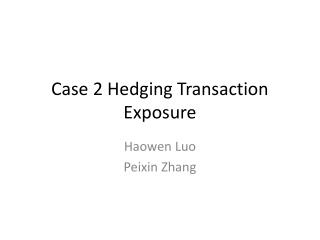DownloadDownload PresentationCase 2 Hedging Transaction Exposure

# Case 2 Hedging Transaction Exposure

Télécharger la présentation## Case 2 Hedging Transaction Exposure

- - - - - - - - - - - - - - - - - - - - - - - - - - - E N D - - - - - - - - - - - - - - - - - - - - - - - - - - -
##### Presentation Transcript

1. Case 2 Hedging Transaction Exposure HaowenLuo Peixin Zhang

2. Story • In June 2009, DW knows that it will have to pay JPY 200,000,000 for the Japanese parts in the future. • Delivery expected to be in October 17, payment is due within 30 days of delivery, or before November 17.

3. Measuring the TE • Net Transaction Exposure (NTE) Spot rate = 0.009829 USD/JPY Net cash outflow = JPY 200,000,000 NTE= JPY 200M*0.009829 USD/JPY = USD 1.9658M

4. Range Estimates • Ad-hoc Analysis USD 1,965,800 (1.10) = USD 2,162,380 USD 1,965,800 (1-10) = USD 1,856,108 Therefore, estimated range for NTE is: (USD 1,856,108; USD \$2,162,380) Note: DW needs to have in the bank USD 2,162,380 to cover the JPY outflow.

5. Range Estimates • Sensitivity Analysis • Simulation (i) convert monthly return to 4-month by using s(t) = [S(t)-S(t-4)]/S(t-4) (ii) Randomly pick 50% samples(240 observations) from empirical distribution (iii) Calculate S(t+4) for each sample that we selected in previous step and calculate TE for each S(t+4) (iv) Plot the TEs in a histogram to construct simulated TE distrubution)

6. Range Estimates • Based on the simulated distribution, we construct a 95% confidence interval. Therefore, the estimated range using simulation is: (\$1,858,818 \$2,096,489) Note: DW needs to have in the bank USD 2,096,489 to cover the JPY outflow.

7. Range Estimates • Sensitivity Analysis • Standard statistical theory • Assumption: Assume that 1-month exchange rate changes follow a normal distribution with mean of 0.0036 and monthly variance of 0.001085, where the man and variance are estimated using the past 39 years of monthly percentage changes. That is st ~ N(0.0036 , 0.001085)

8. Range Estimates • Sensitivity Analysis • Standard statistical theory • Based on the normal distribution, we construct a 95% confidence interval. Therefore, the estimated range using simulation is: (\$1,814,821 \$2,173,736) Note: DW needs to have in the bank USD 2,173,736 to cover the JPY outflow.

9. Value at Risk (VaR) • Input needed to calculate VaR Variance( adjusted to 4-month return) Variance= 4*0.001085 = 0.00434 CI=99% => zα=.01=2.33 Var(99%) = JPY 200M*0.009829 SD/JPY *sqrt(0.00434)*2.33 = = USD 301,745.02 => 4-month worst move is USD 301,745.02

10. PHLX Options • One option contract in PHLX covers 1,000,000 JPY. Therefore, in order to fully cover our position we need 200,000,000/1,000,000=200 contracts.

11. Hedging using PHLX options

12. Hedging using PHLX options

14. Recommendation Based on our estimated TE, we would recommend to use the forward to hedge. It is more likely that the future spot rate will be lower than the strike price. When the future spot rate lower than the strike price, the forward strategy clearly dominate other two hedging strategies.

15. Story On November 6, the Japanese parts arrived on October 11 and payment is due in five days. The exchange rate is 0.008985 USD/JPY. The 1-mo. And 3-mo. Forward USD/JPY rates are 0.0089845, and 0.008985, respectively. U.S. Short interest rates for two months or less are 0.2909-0.3165. The CME Dec futures trades at 0.008987.The PHLX Dec options have the following prices (in U.S. cents):

16. Three Months Forward

17. Six Months Forward

18. Six Months Forward

19. Using Dec Futures

20. Using the OTC JPY Option

21. Using the OTC JPY Option

22. Using JPY Dec Option

23. Left the Position Open

24. Summary